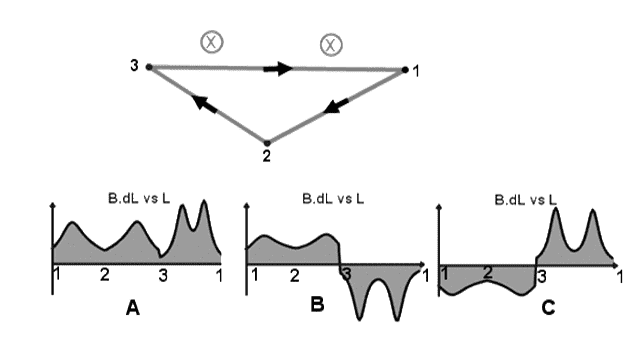# Plots of B•dl as a function of position along the closed path

## Homework Statement

Two infinitely long current carrying wires run into the page as indicated. Consider a closed triangular path that runs from point 1 to point 2 to point 3 and back to point 1 as shown.
Which of the following plots best shows B•dl as a function of position along the closed path?http://i.imgur.com/J7b5L.png

miu*I= B•dl

## The Attempt at a Solution

I used RHR.I am confused about when should it is positive and when is negative.

collinsmark
Homework Helper
Gold Member
I used RHR.I am confused about when should it is positive and when is negative.

Ampère's law states that:

$$\oint _P \vec B \cdot \vec{dl} = \mu_0 I_{enc}$$
The left is a closed path integral. In other words, assuming that the path is closed, the total area under the curve of $\vec B \cdot \vec{dl}$ is proportional to the current enclosed within the path. How much current is enclosed within the path? That should rule out one of the choices right there.

Pick a path component and note the direction of $\vec{dl}$ (the corresponding arrow in the figure will show you the direction of $\vec{dl}$). Use the right hand rule to determine the direction of $\vec B$. For that path, is the direction of $\vec B$ in the same general direction of $\vec{dl}$ (making $\vec B \cdot \vec{dl}$ positive)? Or are they generally in the opposite direction (making $\vec B \cdot \vec{dl}$ negative)? As a sanity check, repeat for the other path components.

Ampère's law states that:

$$\oint _P \vec B \cdot \vec{dl} = \mu_0 I_{enc}$$
The left is a closed path integral. In other words, assuming that the path is closed, the total area under the curve of $\vec B \cdot \vec{dl}$ is proportional to the current enclosed within the path. How much current is enclosed within the path? That should rule out one of the choices right there.

Pick a path component and note the direction of $\vec{dl}$ (the corresponding arrow in the figure will show you the direction of $\vec{dl}$). Use the right hand rule to determine the direction of $\vec B$. For that path, is the direction of $\vec B$ in the same general direction of $\vec{dl}$ (making $\vec B \cdot \vec{dl}$ positive)? Or are they generally in the opposite direction (making $\vec B \cdot \vec{dl}$ negative)? As a sanity check, repeat for the other path components.

So the direction of path is same as direction of current?
Thanks!

collinsmark
Homework Helper
Gold Member
So the direction of path is same as direction of current?
Thanks!
Um no. :uhh: The direction of the current is into the board/paper, as indicated by the 'x's on the wires. The direction of $\vec{dl}$ of each path segment is shown by the arrows on each path segment. (All of that is given to you in the problem statement.) You can determine the direction of $\vec{B}$ by using the right hand rule. (Hint: the direction of $\vec{B}$ is perpendicular to the current in the infinitely long wires. But you need to think in three dimensions.)